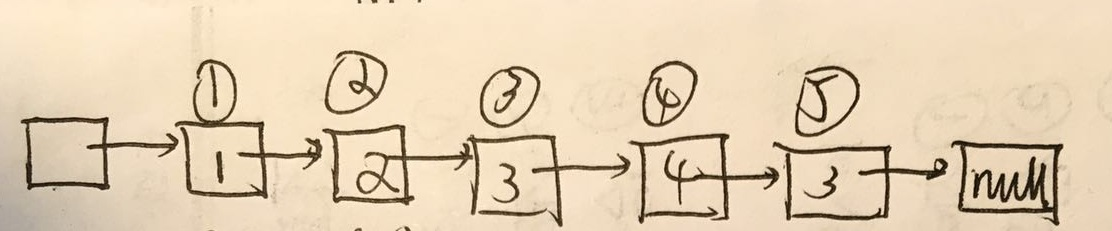`````` void CreateListR(ListNode* &L, int a[], int n)
{
L = (ListNode *)malloc(sizeof(ListNode));
L->next = nullptr; //建立空表
ListNode *s;
ListNode *r;  //指向尾端结点
r = L;

for (int i = 0; i < n; i++)
{
s = (ListNode *)malloc(sizeof(ListNode));
s->data = a[i];
r->next = s;
r = s;
}
r->next = nullptr;  //最后将r->next置为nullptr
}
``````

`````` int a = {1,2,3,4,3};
ListNode *L;
CreateListR(L, a, 5);
```````````` int ListLength(ListNode* L)
{
int length = 0;
ListNode *p = L;
while (p->next != nullptr)
{
length++;
p = p->next;
}
return length;
}
``````

1个回答

`如果回答满意，请点下我回答右边的采纳。如果有不明白的请追问。谢谢`gzrjzcx 您好，我知道正常其实是不需要那个不存数据的头结点，但是它的存在还是会对一些操作的判断会简单一些，所以我想我可能还是需要。另外我的代码中L应该就是不带数据的头结点吧？赋值的时候直接是r的next克：r->next = s; 另外我想确定一点的是，遍历的过程中结点next==nullptr就是结束，这里该结点的next是不是还指向一个nullptr的结点（也就是尾结点）？因为我需要读取倒数第k个结点的内容的时候，可能会影响到判断。 谢谢